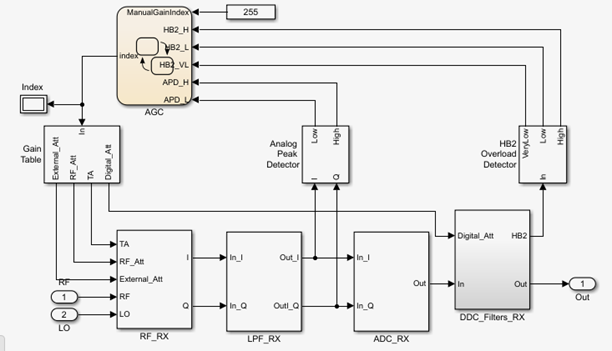You can use the AD9371 models to simulate Analog Devices® AD9371 RF transmitter, receiver, observer, and sniffer designs. These models also helps to see the impact of RF imperfections on your transmitted or received signal.

Install Analog Devices RF Transceivers using `simrfSupportPackages`. You can open models using the Simulink® Library Browser and opening RF Blockset™ Models for Analog Devices RF Transceivers, or by typing the following in the MATLAB® command prompt:

`open ad9371_models`

Choose the model you want from the library:Note

You require these additional licenses to run this model:

• Communications Toolbox™

• Stateflow®

• Fixed-Point Designer™

Complete documentation on how to use the models is available with the software download.

`open(ad93xx_getdoc)`

### AD9371_TX Analog Devices Transmitter

#### Model DescriptionThe transmitter model consists of three stages:

• Digital up-conversion filters (DUC_Filters_TX))

• Analog filters (Analog_Filters_TX)

• RF front end (RF_TX)

You can use the transmitter model to simulate the following behaviors:

• Tunable attenuation

• Attenuation and carrier-frequency dependent noise floor

• Attenuation and carrier-frequency dependent output-referred third-order intercept (OIP3) and output-referred second-order intercept (OIP2)

• Attenuation and carrier-frequency dependent gain imbalance (to model finite image rejection)

• Attenuation and carrier-frequency dependent local oscillator (LO) carrier leakage

#### Model DescriptionThe receiver model consists of three stages:

• RF receiver (RF_RX)

• Analog filters (LPF_RX)

• Analog-to-Digital converter (ADC_RX)

• Digital down-conversion filters (DDC_Filters_RX)

• Receiver signal strength indicator (RSSI): two power meters to detect the strength of the received signal at different section of the chain (Analog Peak Detector, and HB2 Overload Detector)

• Automatic gain control state machine (AGC)

• Programmable gain table (Gain Table)

You can use the receiver model simulate the following behaviors:

• Tunable internal attenuation

• Attenuation and carrier-frequency dependent noise figure

• Attenuation and carrier-frequency dependent output-referred third-order intercept (OIP3) and output-referred second-order intercept (OIP2)

• Attenuation and carrier-frequency dependent gain imbalance (to model finite image rejection)

• Attenuation and carrier-frequency dependent local oscillator (LO) carrier leakage

• Analog filters provide continuous time signal

• ADC models a high-sampling rate third order delta-sigma modulator.

• Digital down conversion digital filters converts the highly sampled signal at the output of the ADC to a lower baseband rate.

• Received signal strength indicator measures power at two stages, at the RF receiver output and after the first half-band filter

• AGC changes the index of the gain table according to the flags of threshold crossing reported by the RSSI.

• Default gain table is read from the MATLAB file, `DefaultGainTables`. You can customize this file.

#### Model DescriptionThe observer receiver model consists of three stages:

• RF receiver (RF_RX)

• Analog filters (LPF_RX)

• Analog to Digital converter (ADC_RX)

• Digital down-conversion filters (DDC_Filters_RX)

• Automatic gain control state machine (AGC) operating in manual control mode

• Programmable gain table (Gain Table)

You can use the observer receiver model to simulate the following behaviors:

• Tunable internal attenuation

• Attenuation and carrier-frequency dependent noise figure

• Attenuation and carrier-frequency dependent output-referred third-order intercept (OIP3) and output-referred second-order intercept (OIP2)

• Attenuation and carrier-frequency dependent gain imbalance (to model finite image rejection)

• Attenuation and carrier-frequency dependent local oscillator (LO) carrier leakage

• Analog filters provide continuous time signal.

• ADC models a high-sampling rate third-order delta-sigma modulator.

• Digital down conversion digital filters converts the highly sampled signal at the output of the ADC to a lower baseband rate.

• Received signal strength indicator measures power at two stages, at the RF receiver output and after the first half-band filter

• Default gain table is read from the MATLAB file, `DefaultGainTables`. You can customize this file.

#### Model DescriptionThe sniffer receiver model consists of three stages:

• RF receiver (RF_RX)

• Analog filters (LPF_RX)

• Analog to Digital converter (ADC_RX)

• Digital down-conversion filters (DDC_Filters_RX)

• Receiver signal strength indicator (RSSI): two power meters to detect the strength of the received signal at different section of the chain (Analog Peak Detector, and HB2 Overload Detector)

• Automatic gain control state machine (AGC) operating in manual control mode

• Programmable gain table (Gain Table)

You can use the AD9371 sniffer receiver model to simulate the following behaviors:

• Tunable internal attenuation

• Carrier-frequency dependent noise figure

• Attenuation and carrier-frequency dependent output-referred third-order intercept (OIP3) and output-referred second-order intercept (OIP2)

• Attenuation and carrier-frequency dependent gain imbalance (to model finite image rejection)

• Attenuation and carrier-frequency dependent local oscillator (LO) carrier leakage

• Analog filters provide continuous time signal.

• ADC models a high-sampling rate third-order delta-sigma modulator.

• Digital down conversion digital filters converts the highly sampled signal at the output of the ADC to a lower baseband rate.

• Received signal strength indicator measures power at two stages, at the RF receiver output and after the first half-band filter

• AGC changes the index of the gain table according to the flags of threshold crossing reported by the RSSI.

• Default gain table is read from the MATLAB file, `DefaultGainTables`. You can customize this file.